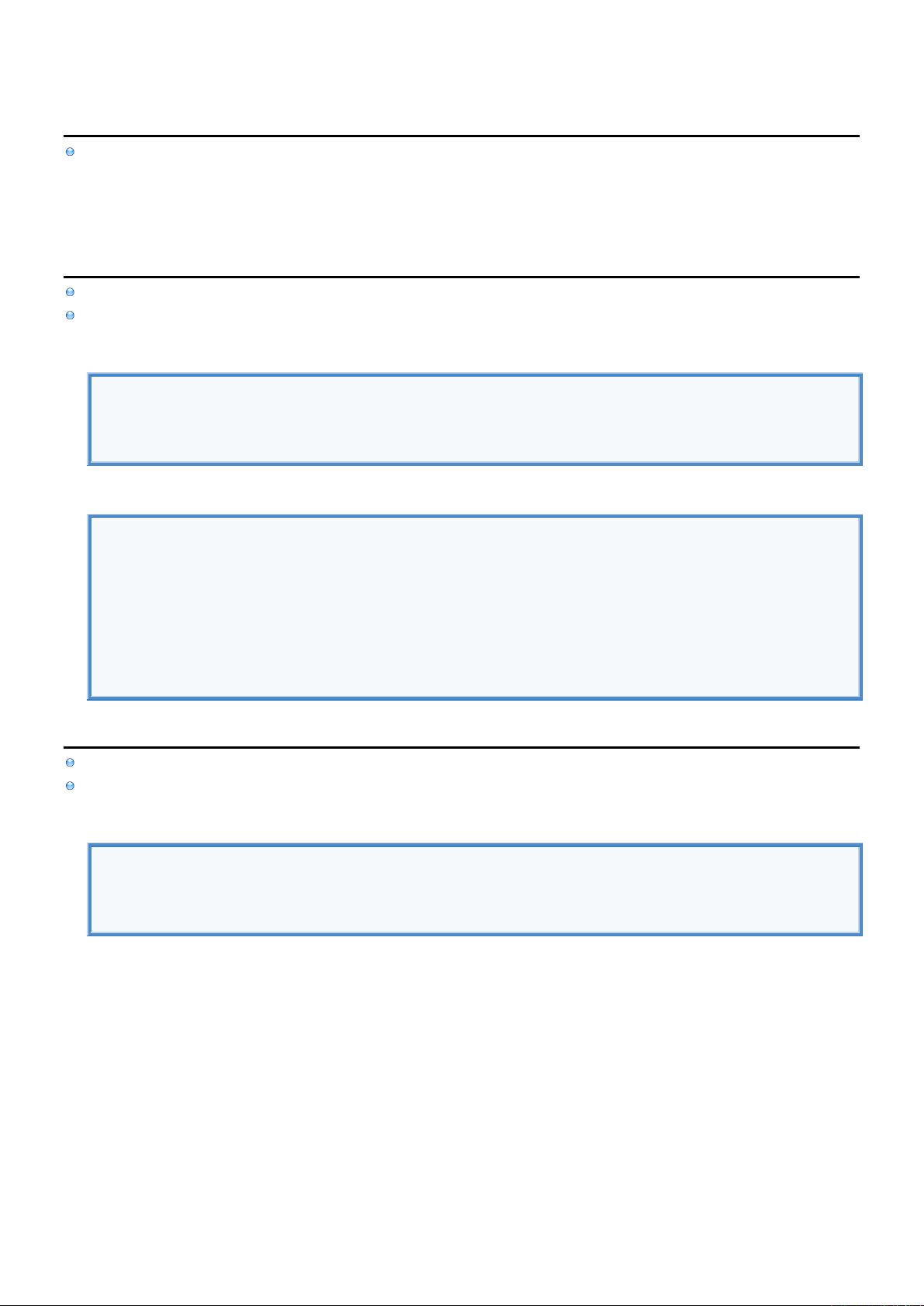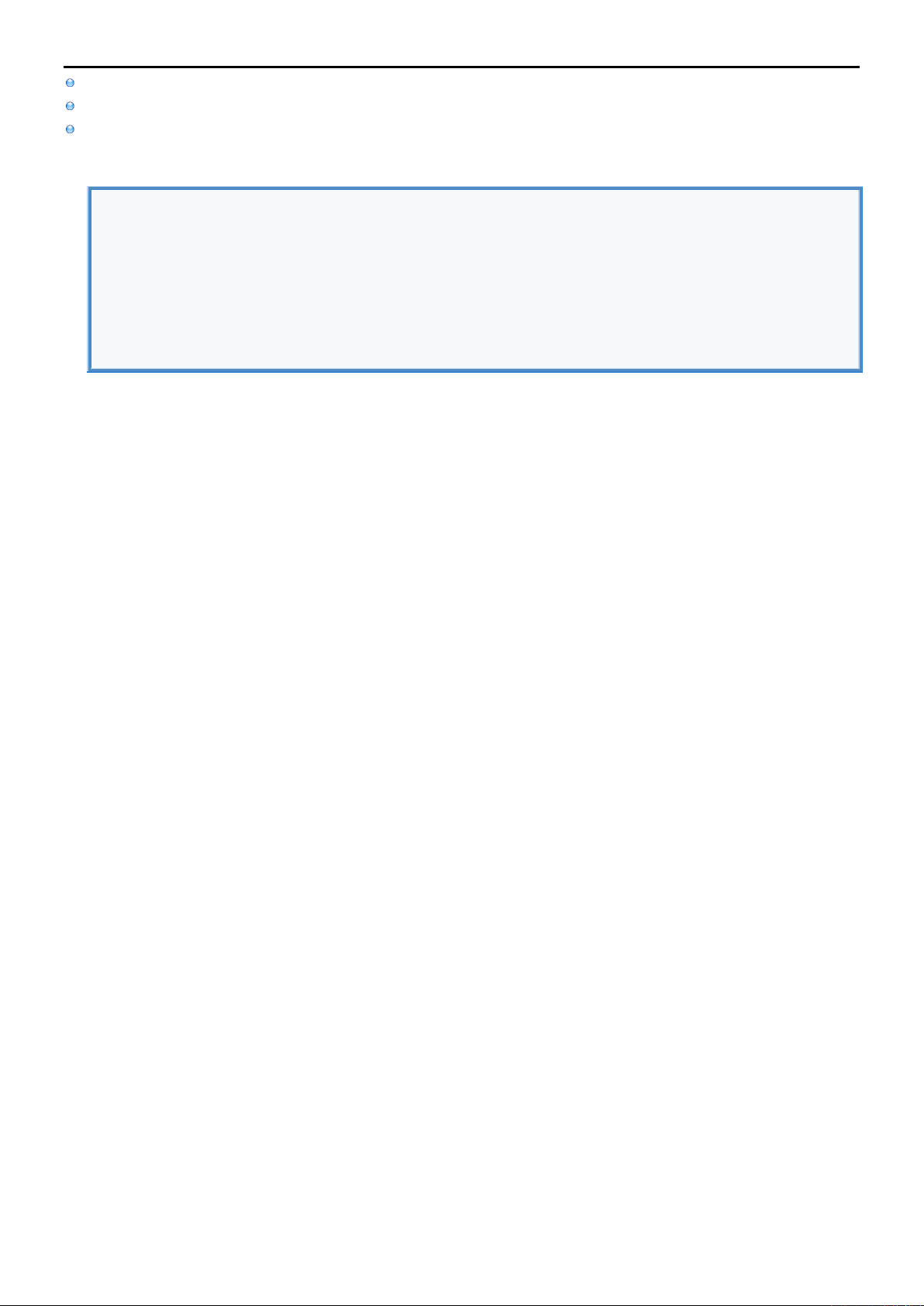2
2
.
.
1
1
.
.
7
7
S
S
t
t
a
a
t
t
e
e
m
m
e
e
n
n
t
t
s
s
-
-
J
J
u
u
m
m
p
p
I
I
n
n
f
f
o
o
Jump Statements are used to skip execution of subsequent code
break completely exits Loop Statement or Switch Block
continue skips execution of the remaining current iteration of the Loop Statement (continues with next iteration)
return returns from the function by skipping execution of the remaining function code
b
b
r
r
e
e
a
a
k
k
break statement is used to completely exit Loop Statements and Switch Blocks.
This means that remaining of the current iteration and all subsequent iterations of the Loop Statement are skipped.
For Loop
for i in 1...5 {
if(i==3) { break } //Exit For Loop.
print(i) //1, 2
}
Switch
var i = 1
switch (i) {
case 1:
print("CASE 1") //Only selected case is executed.
break //Exit Switch.
print("Skipped line")
default:
print("CASE Default")
}
c
c
o
o
n
n
t
t
i
i
n
n
u
u
e
e
continue statement is used to skip only the remaining of the current iteration of the Loop Statement.
But subsequent iterations of the Loop Statement are to be executed normally.
For Loop
for i in 1...5 {
if(i==3) { continue } //Skip next print(i)
print(i) //1, 2, 4, 5
}r
r
e
e
t
t
u
u
r
r
n
n
return statement is used to exit from the function. Code execution continues from where the function was called.
It can also be used to skip execution of the remaining function code.
return statement must be followed by the value if Function should return value.
Return Value
//DECLARE FUNCTION.
func test() -> String {
return("Hello from test.") //Return string.
print("Never executed") //Skipped.
}
//CALL FUNCTION.
var result = test() //Store function return value into variable result.
print(result)